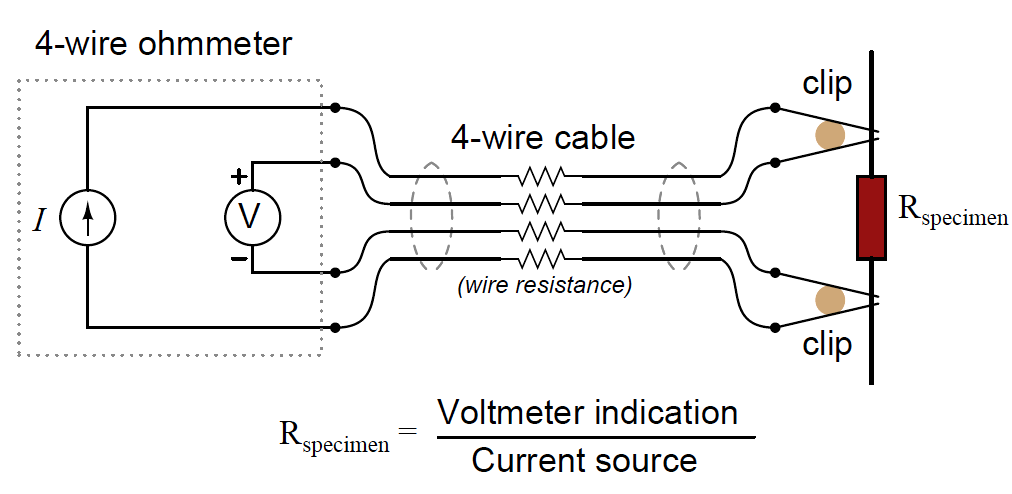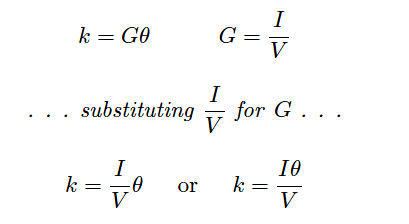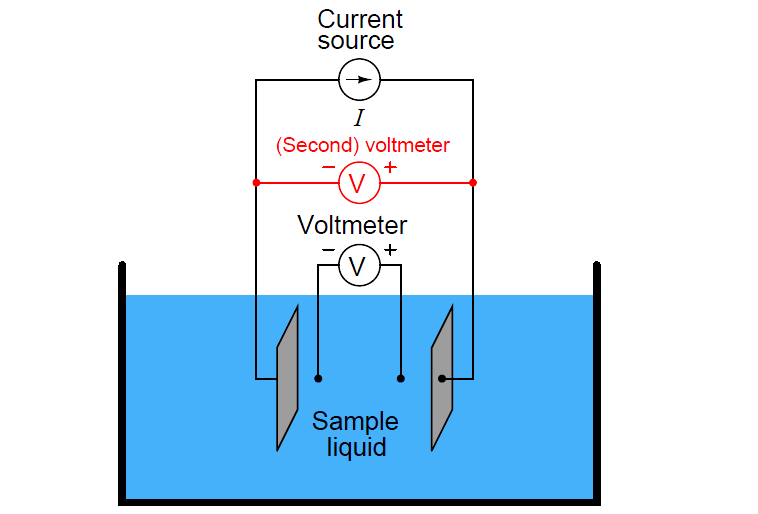# Four Electrode Conductivity Probes Principle

A very old electrical technique known as the Kelvin or four-wire resistance-measuring method is a practical solution to the problem of electrode fouling faced by two-electrode conductivity probes. Commonly employed to make precise resistance measurements for scientific experiments in laboratory conditions, as well as measuring the electrical resistance of strain gauges and other resistive sensors such as RTDs, the four-wire technique uses four conductors to connect the resistance under test to the measuring instrument:Only the outer two conductors carry substantial current. The inner two conductors connecting the voltmeter to the test specimen carry negligible current (due to the voltmeter’s extremely high input impedance) and therefore drop negligible voltage along their lengths. Voltage dropped across the current-carrying (outer) wires is irrelevant, since that voltage drop is never detected by the voltmeter.

Since the voltmeter only measures voltage dropped across the specimen (the resistor under test), and not the test resistance plus wiring resistance, the resulting resistance measurement is much more accurate than if only two wires were used to connect the test meters to the specimen.

In the case of conductivity measurement, it is not wire resistance that we care to ignore, but rather the added resistance caused by fouling of the electrodes. By using four electrodes instead of two, we are able to measure voltage dropped across a length of liquid solution only, and completely ignore the resistive effects of electrode fouling:In the 4-wire conductivity cell, any electrode fouling will merely burden the current source by causing it to output a greater voltage, but it will not affect the amount of voltage detected by the two inner electrodes as that electric current passes through the liquid. Any fouling that happens to occur on the two inner electrodes is of no effect to our conductivity measurement because these inner electrodes carry negligible current. With little or no current through the inner electrodes, there will be negligible voltage dropped across any resistive coating that happens to form on them, and thus the voltmeter will still register the true voltage dropped by the liquid solution.

If the solution’s conductivity is defined as the product of the measured conductance and the cell constant (k = Gθ), and conductance is defined as the ratio of current to voltage (G = I/V ), then we may determine conductivity from voltage and current measurements by combining these two equations:Some conductivity instruments employ a second voltmeter to measure the voltage dropped between the “excitation” electrodes, to indicate electrode fouling:Any form of electrode fouling will cause this secondary voltage measurement to disproportionately exceed the first, thus providing an indicator that instrument technicians may use for predictive maintenance (telling them when the probes need cleaning or replacement). Meanwhile, the primary voltmeter will do its job of accurately measuring liquid conductivity so long as the current source is still able to output its normal amount of current.

Also Read : Toroidal Conductivity Sensors Principle